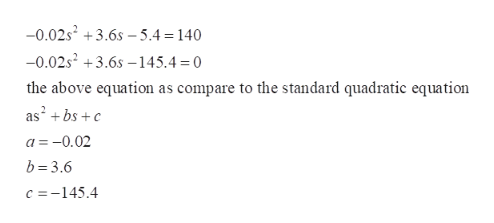# Use the equation with e = 140 to calculate two possible s values using the equation e=-0.02s^2+3.6s-5.4 (round to the nearest whole number) Hint: s=(-b±√(b^2-4ac))/2a

Question
2 views

Use the equation with e = 140 to calculate two possible s values using the equation e=-0.02s^2+3.6s-5.4 (round to the nearest whole number) Hint: s=(-b±√(b^2-4ac))/2a

check_circle

Step 1

According to the provided information,

Step 2

The root of the quadratic equati...help_outlineImage Transcriptionclose-0.02s +3.6s – 5.4 = 140 -0.02s +3.6s -145.4 = 0 the above equation as compare to the standard quadratic equation as' + bs +c a = -0.02 b = 3.6 c =-145.4 fullscreen

### Want to see the full answer?

See Solution

#### Want to see this answer and more?

Solutions are written by subject experts who are available 24/7. Questions are typically answered within 1 hour.*

See Solution
*Response times may vary by subject and question.
Tagged in

### Other